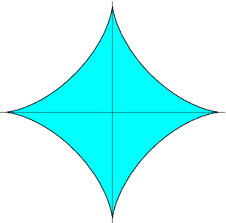## Friday, June 2, 2017

### Unizor - Definite Integrals - Length of Curve

Notes to a video lecture on http://www.unizor.com

Definite Integrals -
Length of Curve

Our task is to calculate a length of a curve on a coordinate plane, defined parametrically as a set of points (x(t),y(t)), where x(t) and y(t) are smooth functions of parameter t∈[a,b].

This is one of the typical integration problem and can be approached similarly to our approach to calculate the area under a curve.

Let's break down a segment [a,b] into N intervals by points a=t0, t1,...tN=b, which results in corresponding breaking of our curve into smaller pieces by points
(x0,y0)=(x(t0),y(t0)),
(x1,y1)=(x(t1),y(t1)),...
...
(xN,yN)=(x(tN),y(tN)),

Each small piece of a curve can be approximated with a straight line from one of its ends to another, and this approximation will be better with the density of break points increasing.
So, the n-th piece of a curve is approximated with a segment from point (xn−1,yn−1) to point (xn,yn).

The length of this n-th piece of a curve Ln can be calculated using the regular formula of a length of a segment between two points on a plane:
Ln² = (xn−xn−1)²+(yn−yn−1

Taking into account that both coordinates are functions of parameter t, we can express it differently:
Ln² = (x(tn)−x(tn−1))² +
+ (y(tn)−y(tn−1))²

Notice that
x(tn)−x(tn−1) ≅ xI(tn)·(tn−tn−1)
where xI(t) is a derivative of function x(t) by t and approximation gets better and better as we increase the density of points tn.

So, we can express the length of the n-th piece of a curve as
Ln² ≅ [xI(tn)² + yI(tn] ·
· (tn−tn−1

From this we get
Ln ≅ SQRT[xI(tn)² + yI(tn] ·
· (tn−tn−1)

As before, we use Δtn=tn−tn−1.
Now the length of a curve can be approximated by this sum:
L ≅ Σn∈[1,N] Ln ≅
≅ Σn∈[1,N] f(xn)·Δtn
where
f(t)=SQRT[xI(t)² + yI(t)²]

Recall the definition of the definite integral:
abf(x) dx =
lim
Σi∈[1,N] f(xiΔxi
where Δxi=xi−xi−1 represents partitioning of segment [a,b] into N parts, and it is assumed that the widest interval Δxi is shrinking to zero by length as N→∞.

Clearly, we are dealing with an integral in our case. The sum of pieces of our curve represents Riemann sum, and the limit of this sum, as the density of points tn increases, is the following integral:
abSQRT[xI(t)² + yI(t)²dt

So, the length of the curve on a coordinate plane, defined parametrically by coordinate functions x(t) and y(t), where t∈[a,b], equals to
abSQRT[xI(t)² + yI(t)²dt

Let's apply this to a couple of practical problems.

Problem 1
Calculate the length of a circle of radius R.

Solution
To define a circle parametrically, let's choose an angle between its radius and a positive direction of the X-axis as a parameter t∈[0,2π].
Then the X-coordinate of a point on a circle, whose radius forms an angle t with positive direction of the X-axis, equals to x(t)=R·cos(t) and Y-coordinate equals to y(t)=R·sin(t).

Now we can use the above formula to calculate the length of circle.
0SQRT[xI(t)² + yI(t)²dt =
0SQRT[R²sin²(t) +
+ R²cos²(t)
dt =
0dt =
R·2π − R·0 = 2πR
As we see, the length, as we counted, equals to the one in a classical formula for the length of the circle. No surprise here.
The end.

Problem 2
Calculate the length of an astroid given parametrically as
x=R·cos³(t)y=R·sin³(t)
where t∈[0,2π]

Solution
Astroid looks like this:Its vertices are at distance R from the origin, and its four arcs correspond to parameter t changing in each quadrant from 0 to .
Using the symmetry, lets' calculate the length of only one arc for t∈[0,π/2] and then multiply it by four.
First, calculate the derivatives by t:
xI(t) = −3R·cos²(t)·sin(t)
yI(t) = 3R·sin²(t)·cos(t)
Now we use the formula for the length of a curve.
SQRT[xI(t)² + yI(t)²] =
3R·|sin(t)·cos(t)| =
(3/2)R·|sin(2t)|
This should be integrated within margins t∈[0,π/2].
Within these margins 2t changes from 0 to π and sin(t) is non-negative, so we can drop absolute value.
So, we need to find the following integral:
0π/2(3/2)R·sin(2t) dt
Indefinite integral of sin(2t) is −cos(2t)/2, which means that we have to calculate the following
(−cos(π)/2) − (−cos(0)/2) =
1/2 + 1/2 = 1.
Therefore, the length of one quarter of an astroid equals to (3/2)R·1 = (3/2)R and the length of an entire astroid is (3/2)R·4=6R.
The end.